# NIUHE

HKUST CSIT5401 Recognition System lecture notes 5. 识别系统复习笔记。

License Plate Recognition is an image-processing technology which is used to identify vehicles by their license plates. This technology is used in various security and traffic applications, such as the access-control system, toll payment, parking fee payment, etc.

A number of license plate recognition units are installed in different locations and the passing vehicle plate numbers are matched between the points. The average speed and travel time between these points can be calculated and presented in order to monitor traffic loads. Additionally, the average speed may be used to issue a speeding ticket.

## Automatic Vehicle Identification System

The installation and responses of sensors help to frame a front-view/rear-view of a passing vehicle. Infra-red sensors are used for vehicle sensing.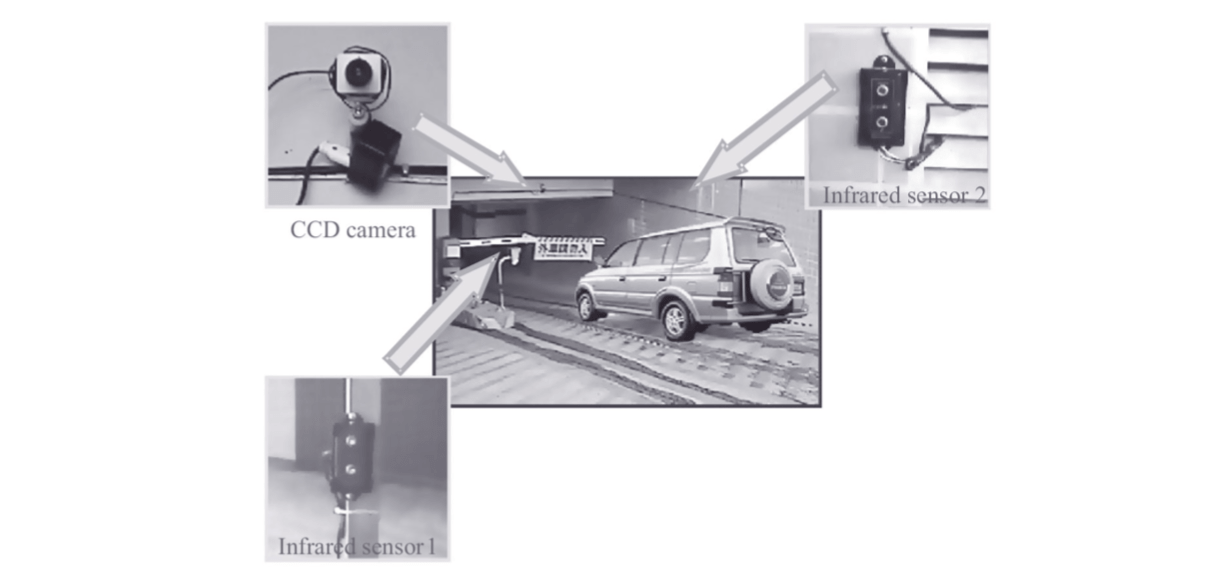Anisotropic magneto-resistive (AMR) sensors for automated vehicle sensing.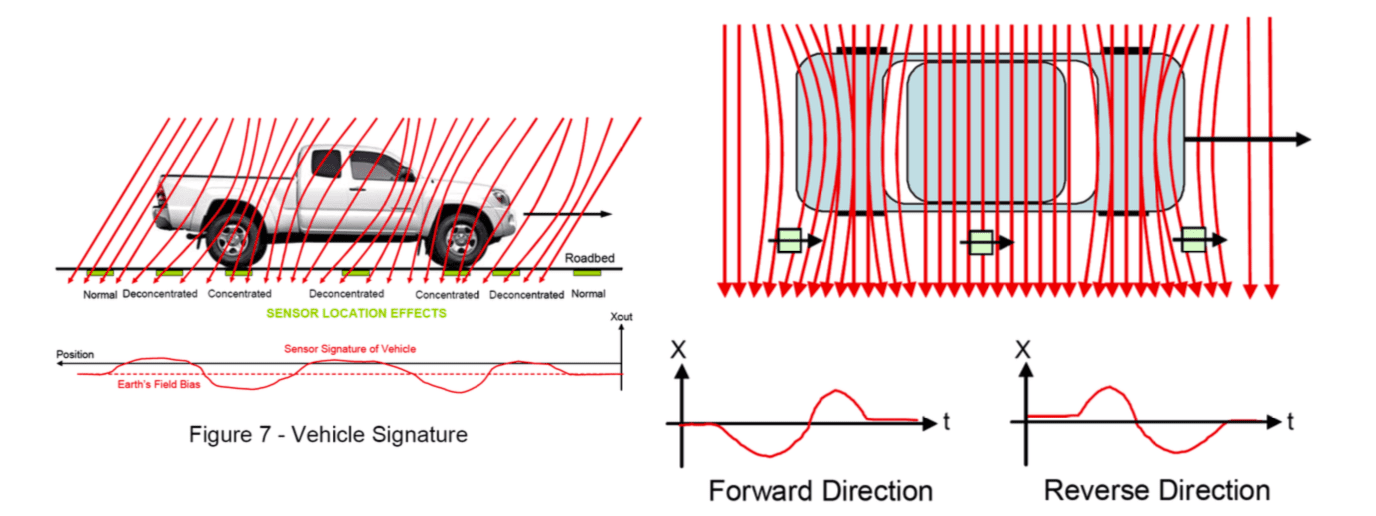License plate recognition is generally composed of three steps.

2. Segmentation of the plate characters (Character Segmentation)
3. Recognition of the plate characters (Character Recognition)

The license plate recognition should operate fast enough to make sure that the system does not miss a single object of interest that moves through the scene.

With the growth of the computer processing power, the latest developments operate within less than 50ms for plate detection and recognition. It enables the processing of more than 20 frames per second for videos.

There are several methods for detecting license plate in a vehicle image.

• Global search [Comelli-TVT-95$]$
• Partial image analysis (vertical edge density)[Anagnostopoulos-TITS-08$]$
• Sliding concentric windows [Anagnostopoulos-TITS-06$]$
• AdaBoost [Dlagnekov-04$]$

### Global Search

Comelli et al. presented a system called RITA. RITA can recognize automatically the characters written on the license plate placed on the rear-side of motor vehicles. The goal is to read only the Italian license plates and reject all the others. It is assumed that a Italian license plate is rectangular and the plate contains black characters over a white background.

The license plate detection algorithm is a global searching method because the algorithm picks within the vehicle image globally the area presenting the maximum local contrast based on gradient analysis. The picked area possibly corresponds to the rectangle that contains the license plate.

The algorithm selects the area that presents the maximum local contrast that (possibly) corresponds to the rectangle that contains the license plate.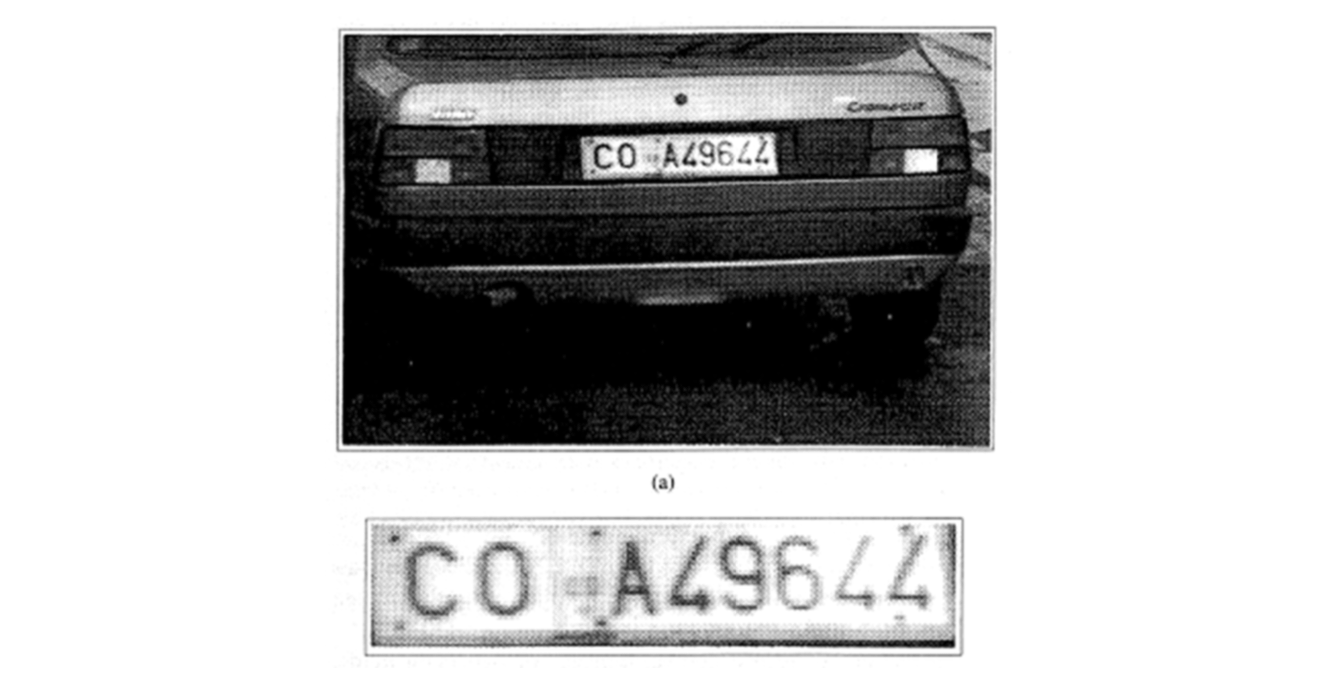### Partial Image Analysis

The vehicle image can be filtered to extract vertical edges and scanned with N-row distance. The number of the existing edges along each scan line is recorded.

If the number of the edges is greater than a threshold value, the presence of a plate can be assumed.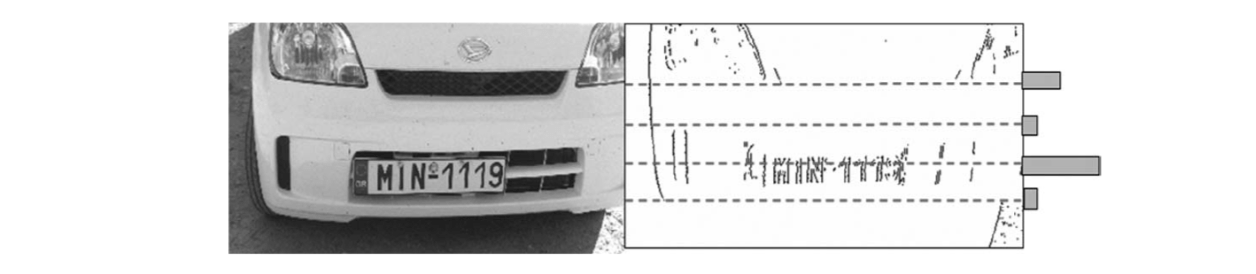Specifically, if the plate is not found in the first scanning processing, then the algorithm is repeated, reducing the threshold for counting edges or adjusting the threshold for finding vertical edges.

### Sliding Concentric Windows

An adaptive image segmentation technique, called sliding concentric windows (SCW), was proposed for license plate detection. The SCW method was developed to describe the local irregularity in the vehicle image.

The method uses image statistics such as the standard deviation and the mean for finding possible plate locations.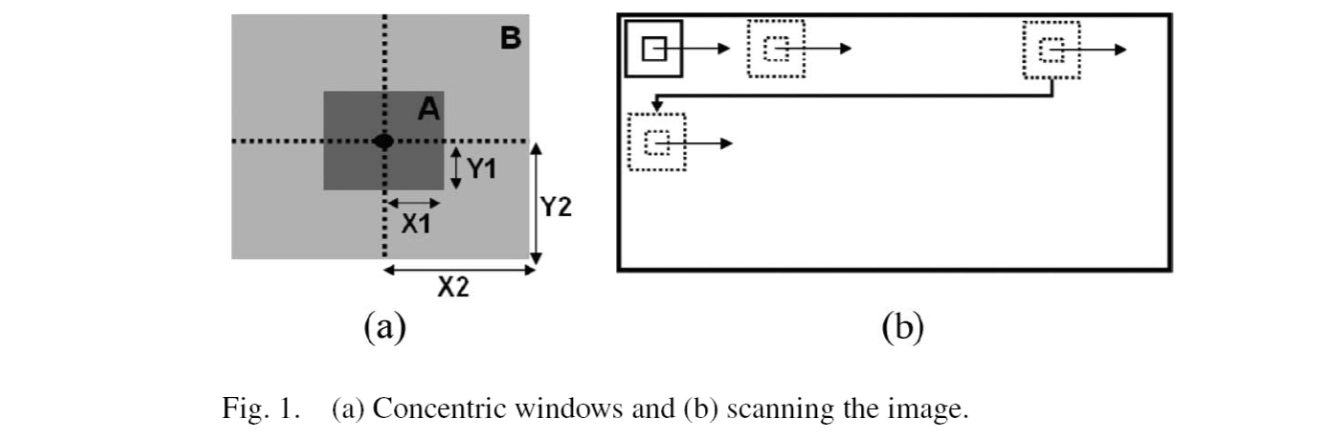In two concentric windows A and B of different sizes ($2X_1\times 2Y_1$ and $2X_2\times2Y_2$ respectively), which scan the vehicle image from left to right and from top to bottom, the mean or the standard deviation is calculated.

If the ratio of the statistical measurements in the two windows exceeds a threshold set by the user, then the central pixel of the concentric windows is considered to belong to a license plate. $I_{output}=\begin{cases} 0 & \text{if }\frac{M_B}{M_A}\leq T,\\ 1 & \text{if }\frac{M_B}{M_A}> T \end{cases}$ where $M$ is the statistical measurement, eigher mean or standard devation.

The result is a binary image $I_{output}$, which eliminates all the redundant regions from the original vehicle image.

The result binary image is used as a mask for highlighting the license plate by computing the product between the binary mask and the input vehicle image. The license plate can then be found in the highlighted image based on the binary mask.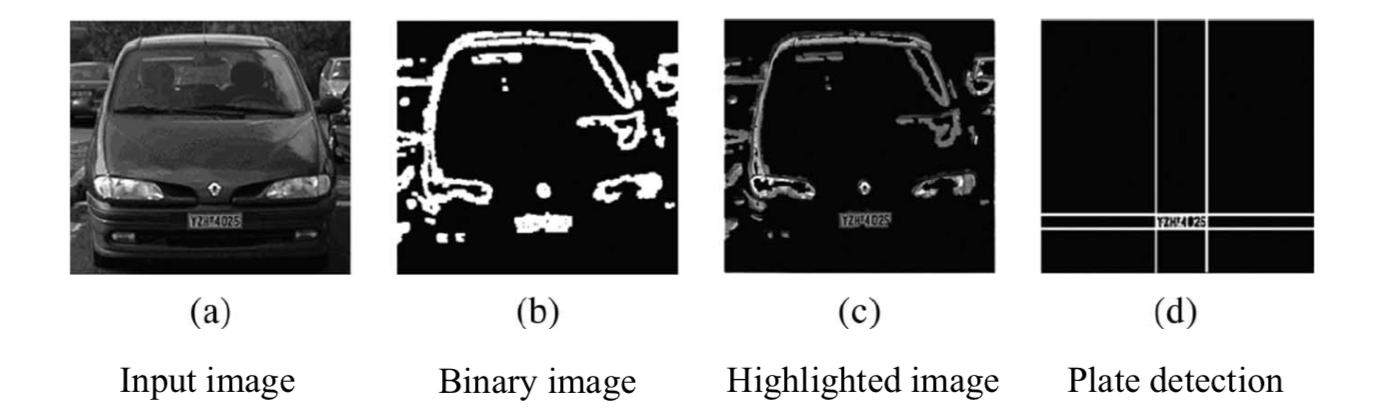Adaptive boosting (AdaBoost) was used in conjunction with the rectangle features for training a strong classifier based on weak classifiers.

For detecting license plates, a total of 100 rectangle features can be applied to sub-regions sized 45(columns) × 15(rows) pixels being scanned as the expected license plate areas in the original vehicle image.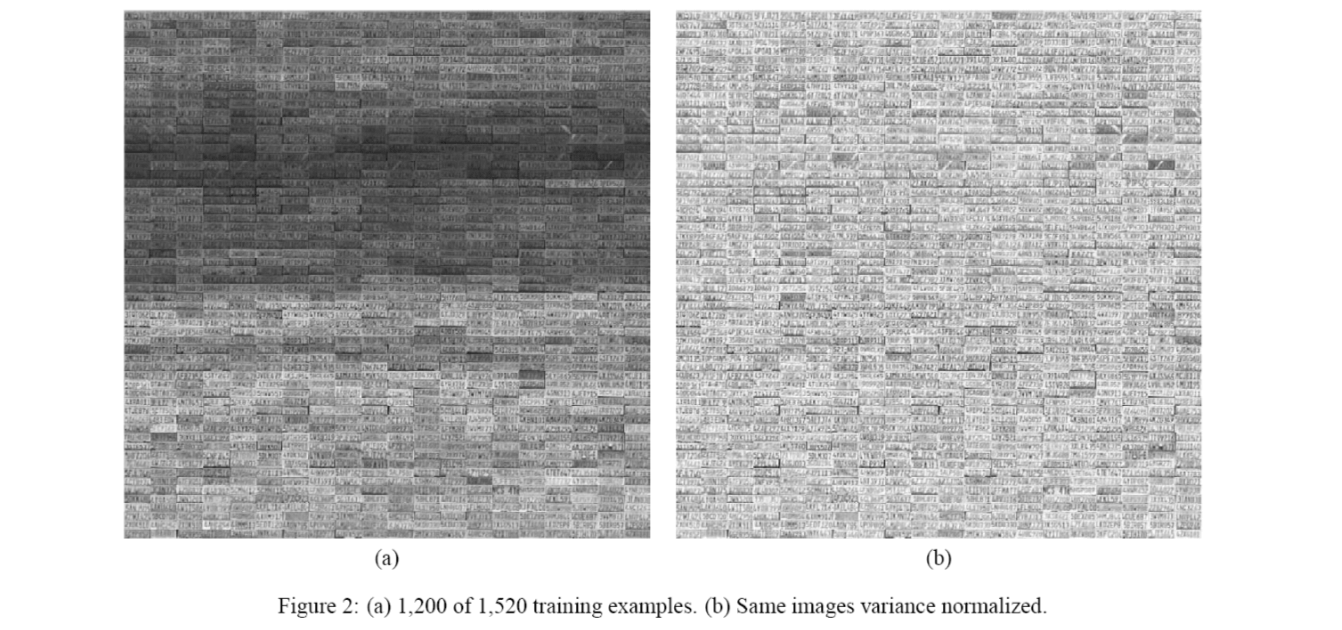Within the 100 rectangle features for detection, there are 37 variance based features, 40 x-derivative features, 18 y-derivative features, and 5 mean pixel intensity features.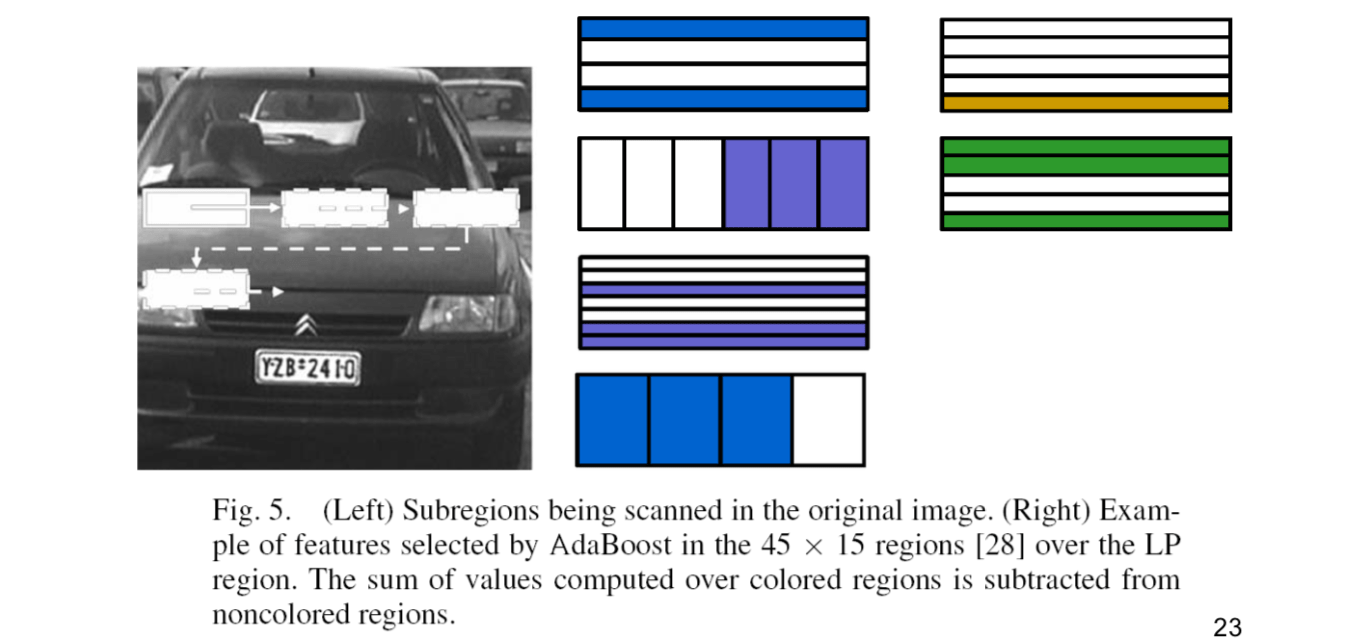When sliding the search window across the vehicle image to be analyzed, several matches can be found. Clustering method can be used to group detected windows that are close to each other and use the mean window as the detected location.

## Character Segmentation

In most systems with a subsequent recognition module, the vertical resolution of the plate vary from 20 to 40 pixels. Prior to character recognition, the detected license plates are enhanced for improving plate image quality, e.g., image normalization and histogram equalization.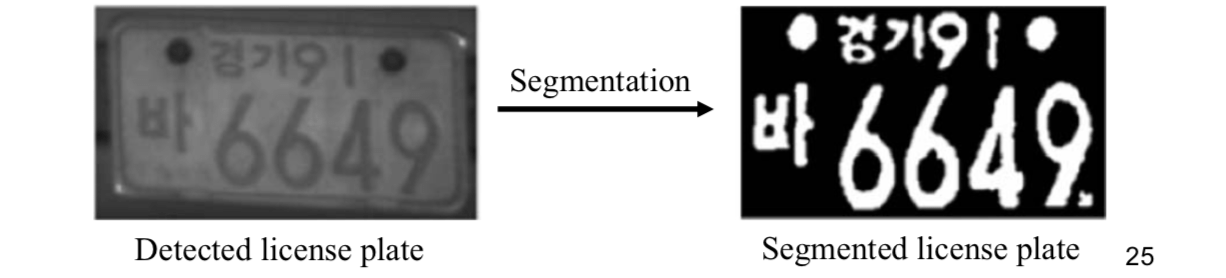Given the enhanced detected license plate image, the goal is to segment each character in the image. A global threshold can be found to segment the detected license plate. Otsu's method is one of widely used methods for image binarization.

Otsu's method

The method is designed for finding optimum global threshold for image binarization and is optimum in the sense that it maximizes the between-class variance.

There are six steps.

1. Compute the normalized histogram of the input image. Denote the components of histogram by $p_i=\frac{n_i}{MN}, i=0, 1, ..., L-1$ where $L$ is the number of gray levels; $n_i$ is the number of pixels with intensity $i$; $M$ is the number of rows; and $N$ is the number of columns.

2. Compute the cumulative sums (the probability that a pixel is assigned to class $C_1$) $P_1(k)=\sum_{i=0}^kp_i$ where $k$ is current threshold for thresholding the input image into two classes $C_1$ and $C_2$.

3. Compute the cumulative means $m(k)=\sum_{i=0}^kip_i,\ k=0,1,...,L-1$

4. Compute the global intensity mean $m_G=\sum_{i=0}^{L-1}ip_i$ where $L$ is the number of gray levels.

5. Compute the between-class variance $\sigma^2_B(k)=\frac{[m_GP_1(k)-m(k)]^2}{P_1(k)[1-P_1(k)]},\ k=0, 1, ..., L-1$

6. Obtain the Otsu threshold, $k^\ast$, as the value for $k$ for which the value of between-class variance is maximum. If the maximum is not unique, obtain $k^\ast$ by averaging the values of $k$ corresponding to the various maxima detected. $\sigma^2_B(k^\ast)=\max_{0\leq k\leq L-2}\sigma^2_B(k)$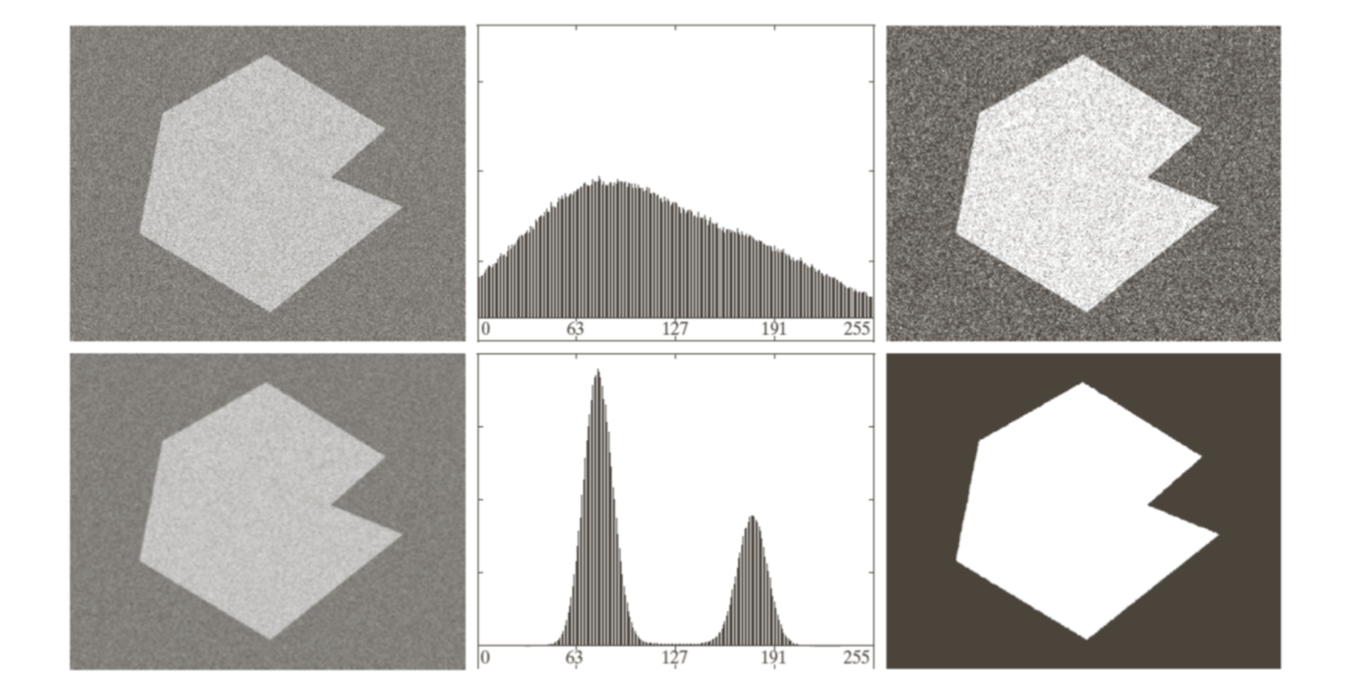Global thresholding on the entire image may not always produce useful results due to uneven lighting environment.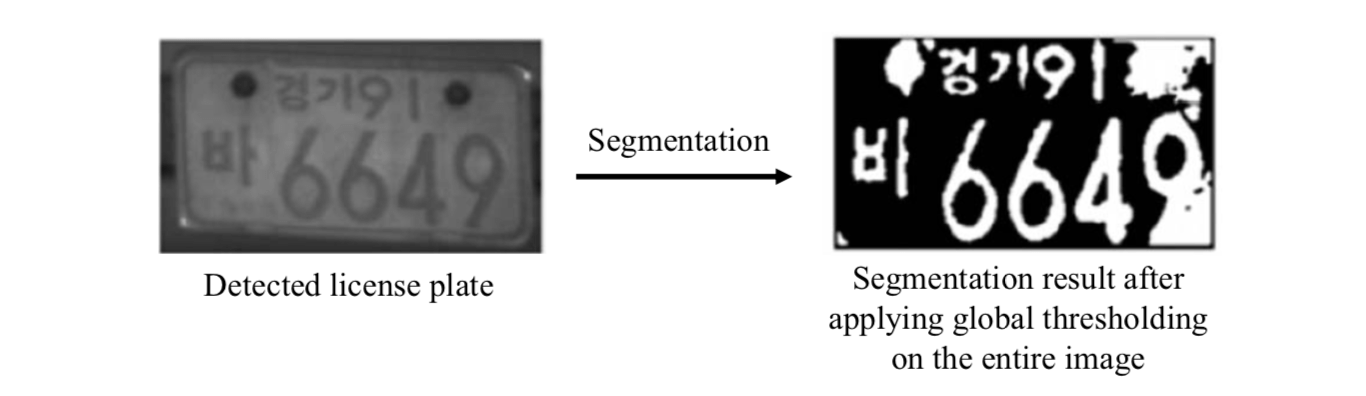Characters can be extracted from the license plate image. Each character can then be segmented by using the thresholding method. Instead of dividing the image into regular blocks, the shape (size) of each block is defined adaptively for each character.Projections of binary edge images are performed. Rows of strings are separated based on the horizontal pixel accumulation. Same for columns of characters.

After the blocks for characters are defined adaptively, the Otsu's method is applied for each blocks adaptively.

Maximally stable extremal regions

Characters can be extracted and segmented by thresholding the image with a variable brightness threshold, and using the enumeration of extremal regions which are stable for a large range of the threshold $T$.

Extremal regions are connected components of an image binarized at certain threshold. When the threshold $T$ is increasing/decreasing, the behavior of the extremal regions is used for character classification and segmentation.

Maximally stable extremal regions (MSERs) are usually of arbitrary shape. The MSER detector is stable and invariant to affine transformations, which is useful for handling viewpoint changes.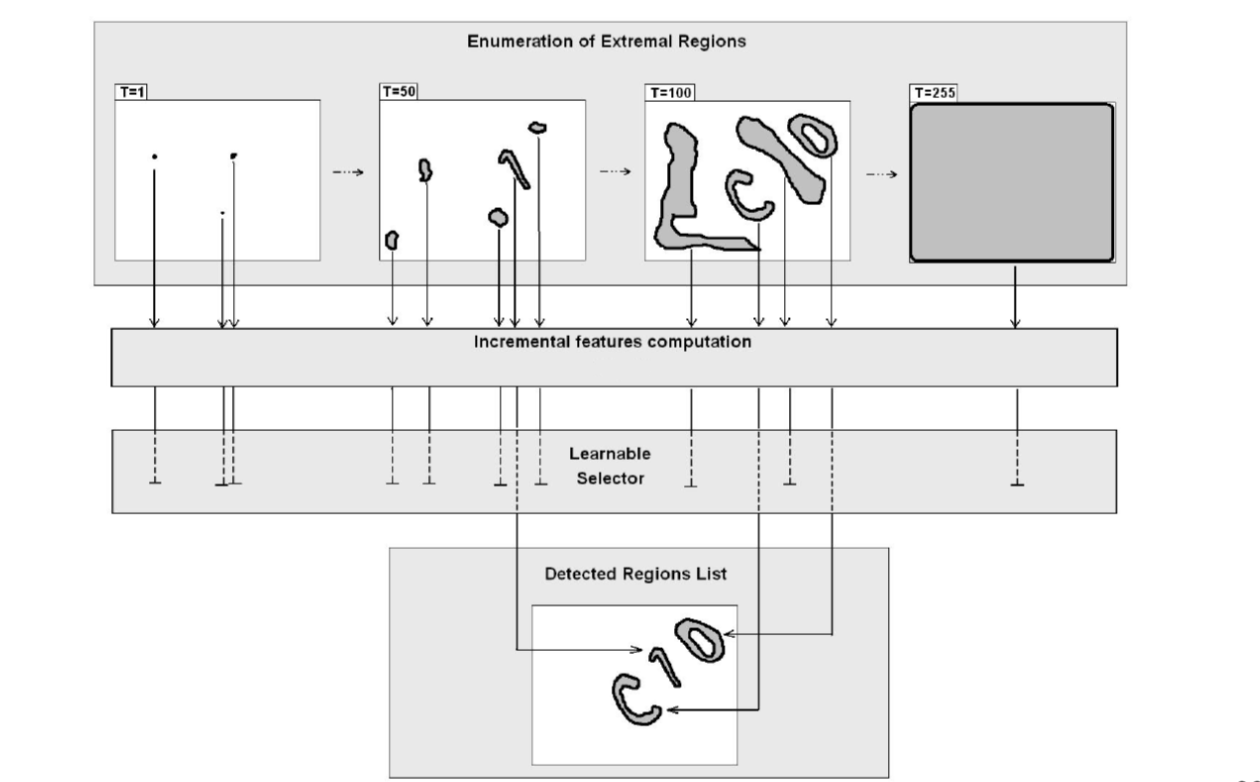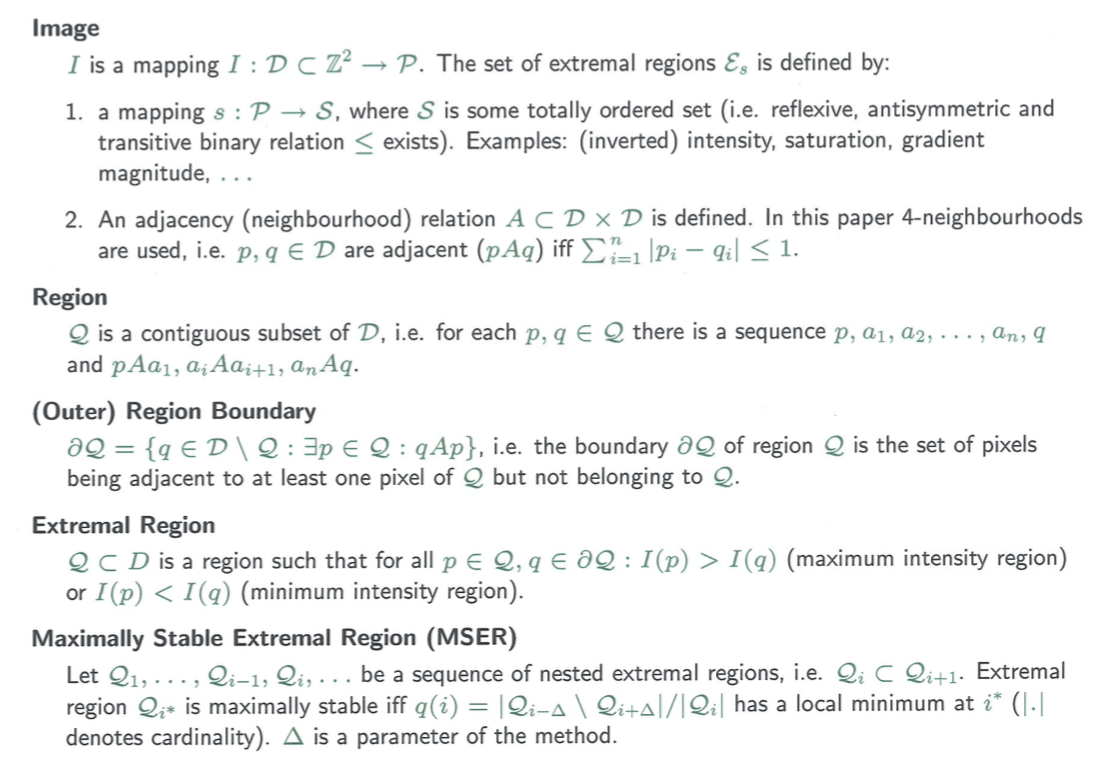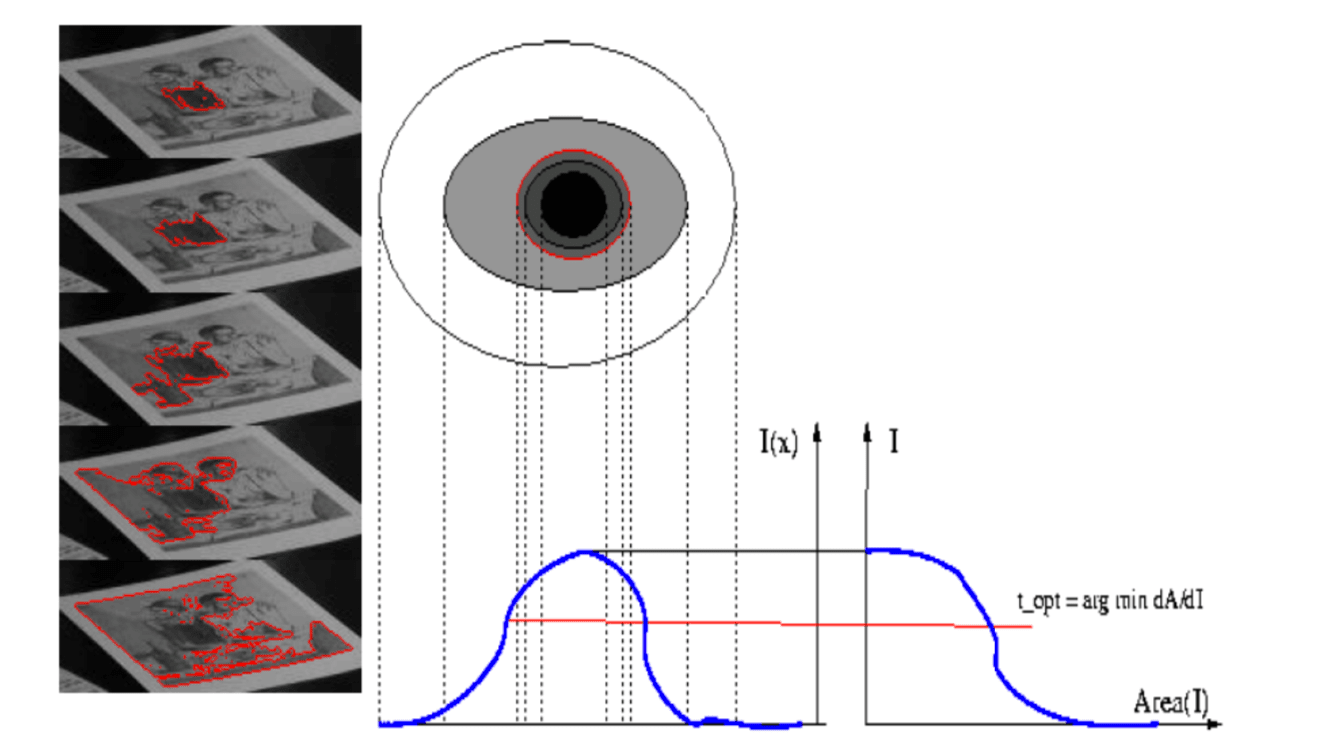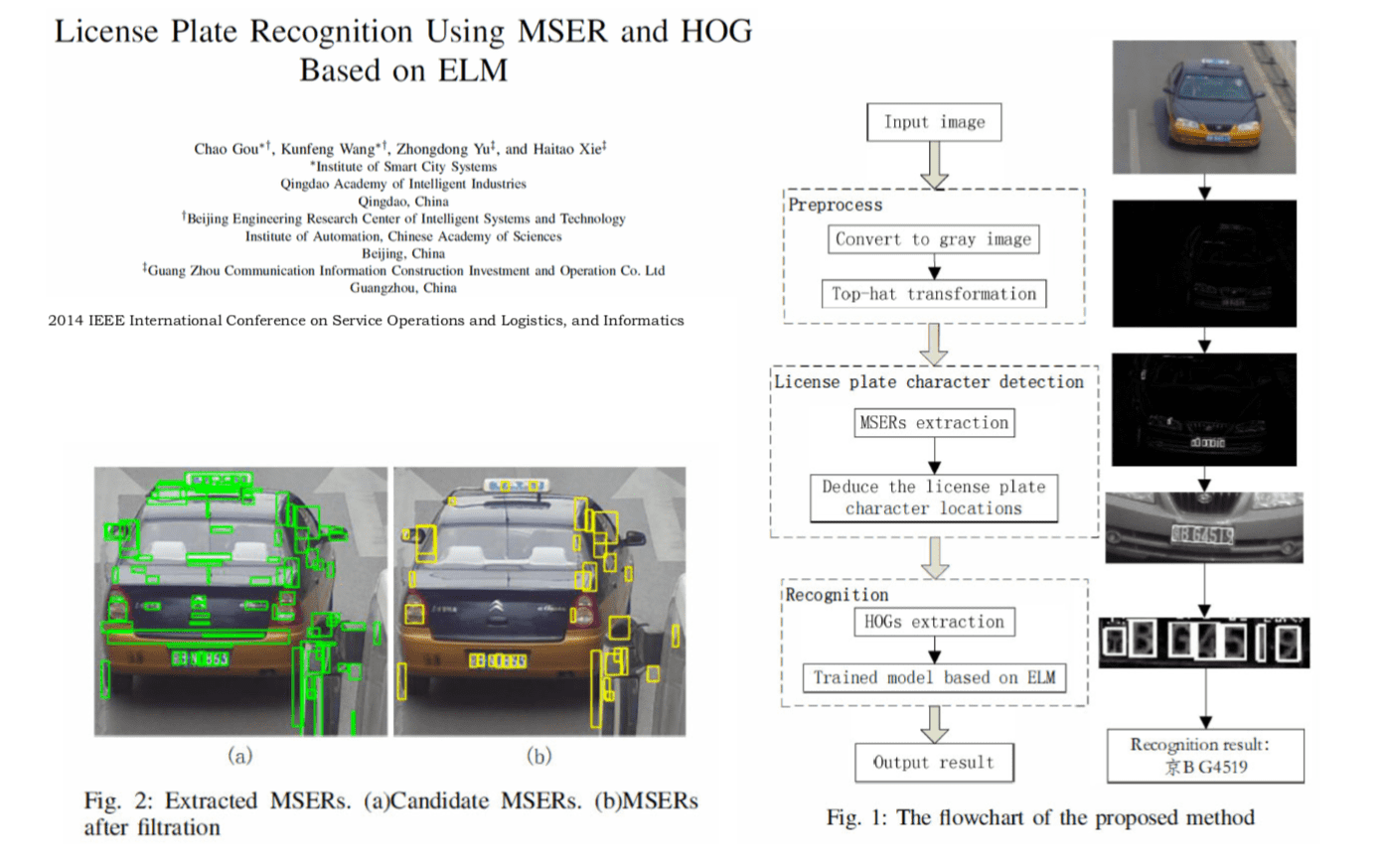## Character Recognition

After the characters are segmented, the segmented characters will be matched against a set of pre-defined characters, e.g. ten numerals (zero to nine), alphabets, etc.

The pre-defined characters usually have single font, fixed character size, and are not rotated heavily. Therefore, pattern/template matching is a suitable technique for character recognition. Templates can be generated in advance for the matching tasks.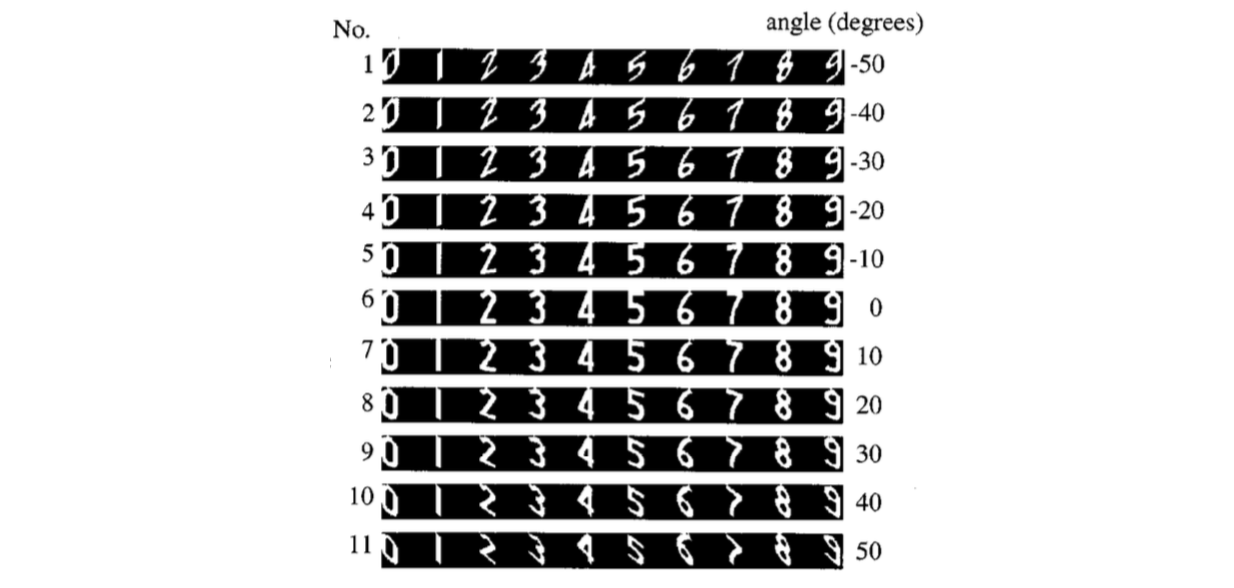The matching process can be done by computing the normalized cross-correlation values for all the translational shifts of each character template over the character block (sub-image).

The normalized cross-correlation is defined as $C_{fg}=\frac{\sum_{m=1}^M\sum_{n=1}^N(f(i,j)-\bar{f})(g(i,j)-\bar{g})}{\sqrt{\sum_{m=1}^M\sum_{n=1}^N(f(i,j)-\bar{f})^2(g(i,j)-\bar{g})^2}}$ where $g$ is shifted template and $f$ is character block.

More advanced techniques, e.g. shape context, can be used for character recognition. ([Belongie-02, Treiber-10])